# Summing Amplifier

This is an example of an inverting amplifier of gain=1 with multiple inputs. More than two inputs can be used, for example in an audio mixer circuit. The input resistors can be unequal, giving a weighted sum.

 Rules application
 Applications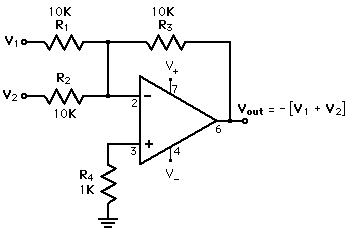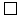Menu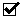Summing amplifierDifference amplifierDifferential amplifier
Index

Electronics concepts

Op-amp concepts

 HyperPhysics*****Electricity and magnetism R Nave
Go Back

# Rule Application:Summing Amplifier

 The behavior of most configurations of op-amps can be determined by applying the "golden rules". For the summing amplifier by the current rule the non-inverting input is a virtual ground. Then the current into A must be zero. This requires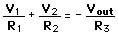Hence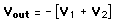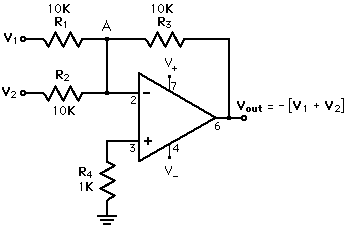Index

Electronics concepts

Op-amp concepts

 HyperPhysics*****Electricity and magnetism R Nave
Go Back

# Applications: Summing Amplifier

The summing amplifier" is a versatile device for combining signals. It can either add signals directly, or scale them to fit some predetermined combination rule.

1. Summing several signals with equal gains is done in an audio mixer.
2. A summing amplifier with different resistors on the inputs gives a weighted sum. This can be used to convert a binary number to a voltage in a digital-to-analog converter.
3. A summing amplifier can be used to apply a DC offset voltage along with an AC signal voltage. This is done in a LED modulation circuit to keep the LED in its linear operating range.
Index

Electronics concepts

Op-amp concepts

 HyperPhysics*****Electricity and magnetism R Nave
Go Back